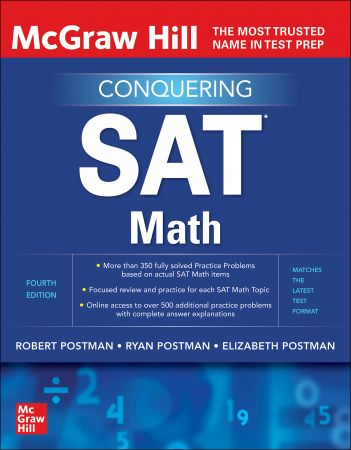McGraw Hill Education Conquering SAT Math, 4th EditionEnglish | December 24th, 2021 | ISBN: 1260462579 | 352 pages | True EPUB | 51.26 MB

The math sections of the SAT are extremely challenging, so we’re here to help. Conquering SAT Math-covering the latest test format-has been specially designed by three math experts who give you strategies for solving every kind of SAT math question. You’ll also get intensive practice for every math question type to help build your test taking confidence. With Conquering SAT Math, you’ll have everything you need to get ready for test day, and achieve your best SAT score.

McGraw-Hill Education Conquering SAT Math includes:
• Hundreds of sample questions with complete answer explanations to maximize your score on the math sections
• Complete review of SAT math topics including: factors, ratios, percents, powers, basic algebra, geometry, functions, probability, and more
• Strategies for answering every SAT multiple-choice and constructed-response math problem
• Drills and exercises to build mathematics problem-solving skills with solved problems of gradually increasing difficulty
• Key test and calculator strategies for answering each SAT Math question type
• Information on the SAT math item formats that reflects the latest test
• Online access to 300 additional practice problems organized by SAT Mathematics Topic and 250 additional practice problems in five SAT Format practice sets